Friday , May 24 2019
Home » Igcse Physics Revision Notes » General Wave Properties

# General Wave Properties

### Wave:

A wave is a phenomenon, in which energy is transferred through vibrations.

### Properties:

• The source of any wave is vibration
• Waves transfer energy from one point to another.
• In waves, energy is transferred without the medium being transferred.

### How Waves Are Formed:

There are two ways to form a wave.

Waves Motion On A Rope:

• Fixing one end of the rope to a wall and moving the other end up and down
• These up and down movements produce vibrations.
• The rope waves travel towards the wall while the rope itself moves up and down.
• The kinetic energy from the up and down movement is transferred from one point to another.
• The rope itself however doesn’t move from one end to another.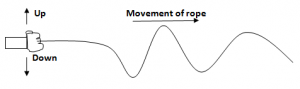### Water Waves In A Ripple Tank:

• Water waves can be generated using a ripple tank
• A small dipper moves up and down
• The water particles at the surface are made to move up and down spreads to other parts of the water surface.
• The kinetic energy is thus transferred to the water molecules at the surface.
• These water molecules in turn transfer to the neighboring water molecules.
• Though energy is passed but the water itself doesn’t move the dripler to the edges.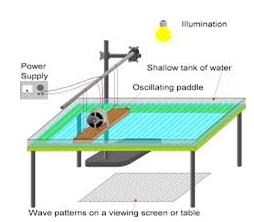Types Of Waves:

Waves are of two types Transverse and Longitudinal.

### Transverse Waves: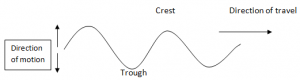• Transverse  waves are waves that travel in a direction perpendicular to the direction of vibration.
• Displacement of particles in transverse waves is perpendicular to the direction of travel of wave motion.
• The highest point reached by a vibrating particle in a transverse wave is called crest or peak while the lowest peak is trough.
• e.g. water, rope, electromagnetic waves

### Longitudinal Waves: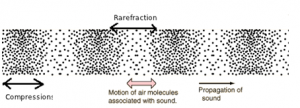• Longitudinal waves are waves that travel in a direction parallel to the direction of vibration.
• Displacement of the particles in longitudinal waves is in line with, or parallel to the direction of wave motion.
• e.g. sound waves

Compression: Section where the vibrating particles in a longitudinal wave are closest together.

Reflection: Section where the vibrating particles are furthest apart.

### Describing Waves: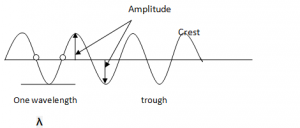Crests and Troughs: These are the highest and the lowest points of a transverse wave, respectively. For longitudinal waves, the terms compression and rarefactions are used.

Phase: Any two points are said to be in phase when they move in the same direction and have the same speed and in the same displacement from the rest position (e.g.      These two points)

Wavelength( λ): This is the shortest distance between any two points in a wave that are in phase such as two successive crests or troughs. The SI unit is metre (m).

Amplitude: This is the maximum displacement from the rest or centre position. It is the height of crest or trough measured from the rest position.

Period (t): Time taken for one point on the wave to complete one oscillation. This can also be written as the time taken, to complete one wave.

SI Unit is seconds(s)

On a displacement time graph one period = 1 sec

Frequency(f): This is the number of complete waves produced per second.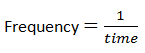The SI unit is Hertz.

Wave Speed (V): The distance of the wave moved in one second in the medium.

V = f  × λ

SI unit: metre per second (ms-1)

Wavespeed = frequency × wavelength

### Wave Front:

It is the imaginary line on a wave that joins all points that are in same phase. It is usually drawn by joining down all the crests.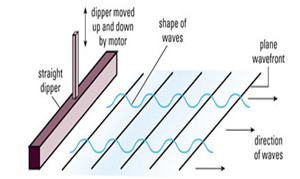Plane dipper can produce plane waves in a ripple tank. Thus plane Wave fronts are seen.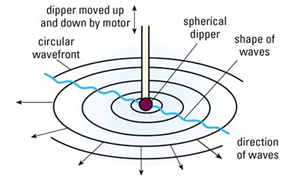Spherical dipper on the other hand can provide circular waves in a ripple tank. These types of waves have circular  Wave fronts.

### About Admin## Mass Weight And Density

Mass: Mass is the measure of amount of substance in a body. (Irrespective of its …